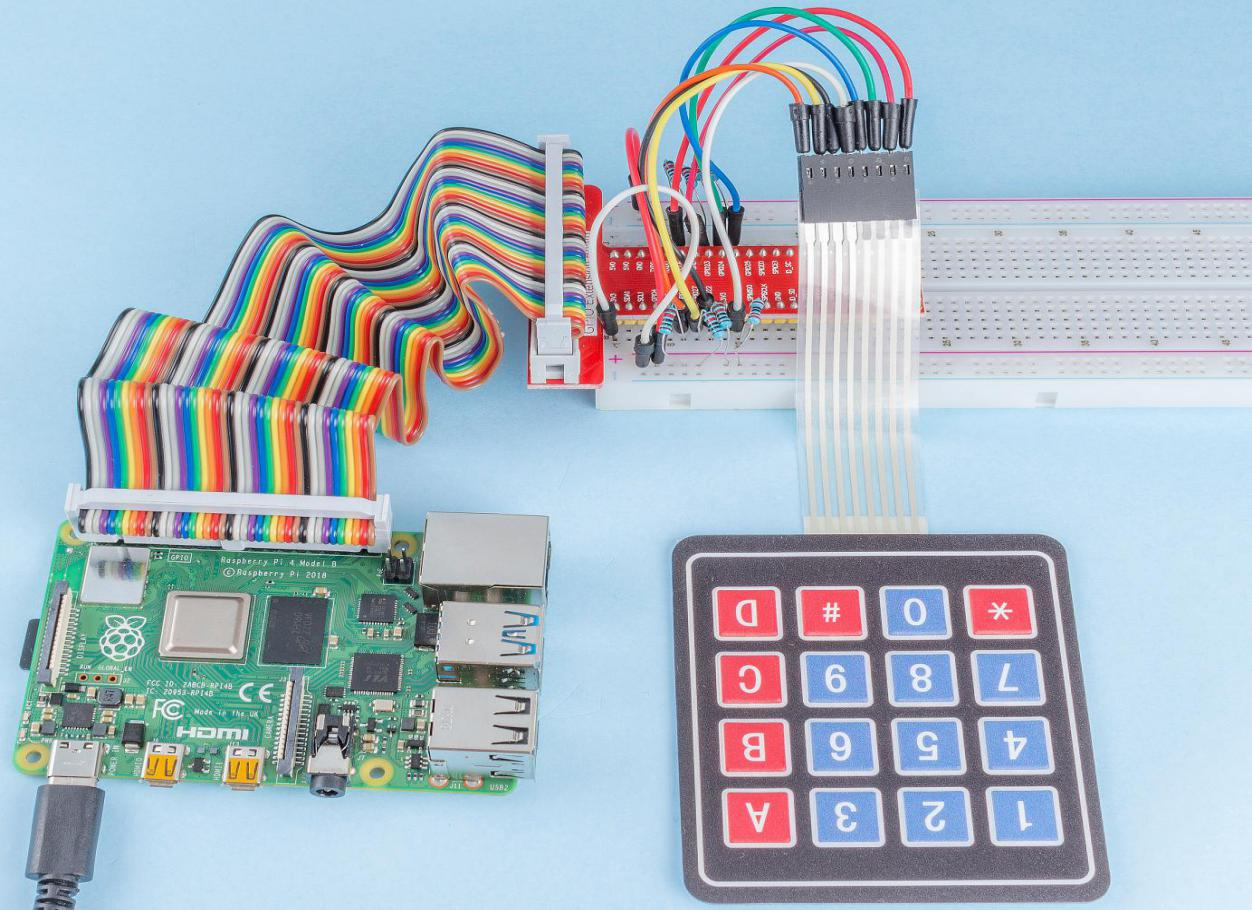## Introduction¶

A keypad is a rectangular array of buttons. In this project, We will use it input characters.

## Components¶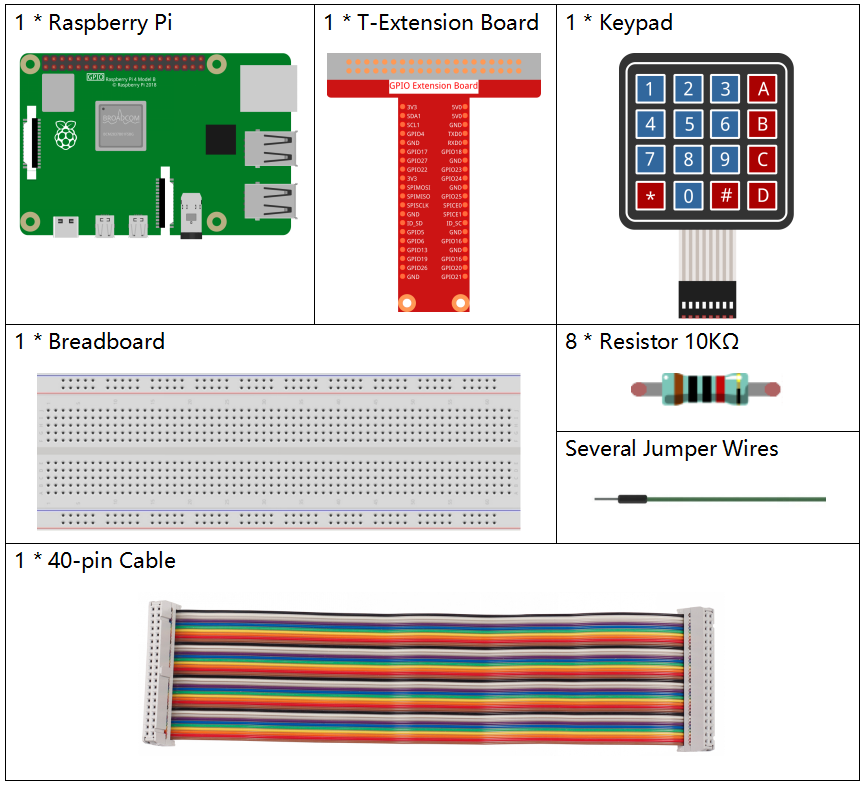## Principle¶

A keypad is a rectangular array of 12 or 16 OFF-(ON) buttons. Their contacts are accessed via a header suitable for connection with a ribbon cable or insertion into a printed circuit board. In some keypads, each button connects with a separate contact in the header, while all the buttons share a common ground.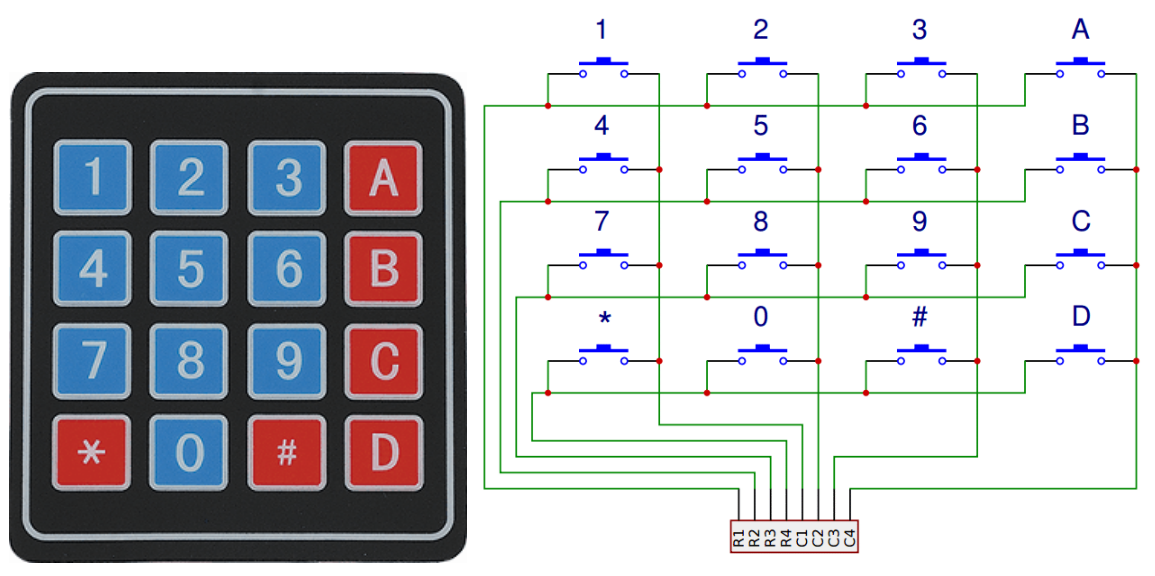More often, the buttons are matrix encoded, meaning that each of them bridges a unique pair of conductors in a matrix. This configuration is suitable for polling by a microcontroller, which can be programmed to send an output pulse to each of the four horizontal wires in turn. During each pulse, it checks the remaining four vertical wires in sequence, to determine which one, if any, is carrying a signal. Pullup or pulldown resistors should be added to the input wires to prevent the inputs of the microcontroller from behaving unpredictably when no signal is present.

## Schematic Diagram¶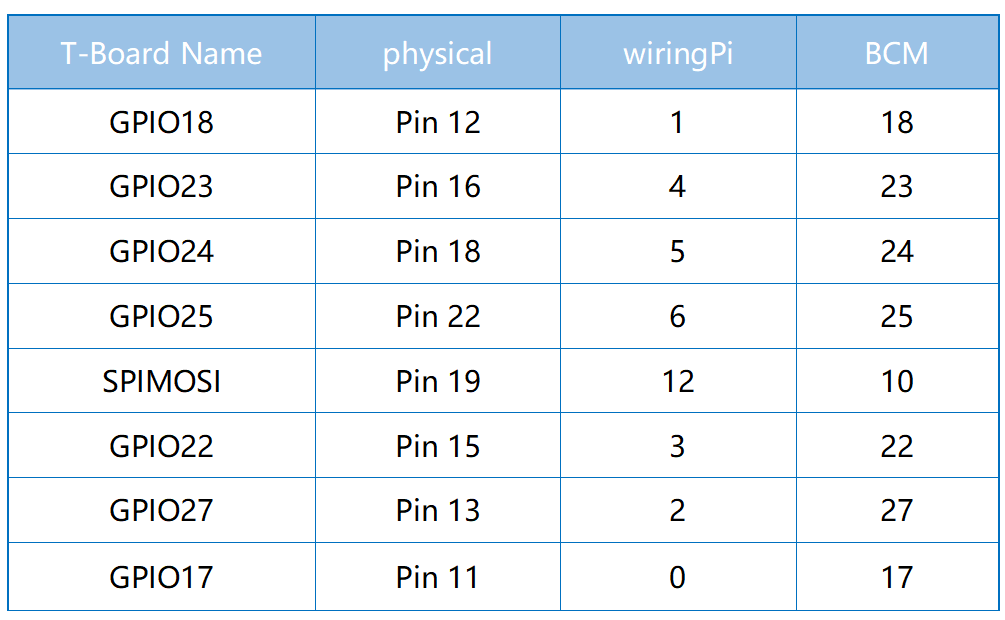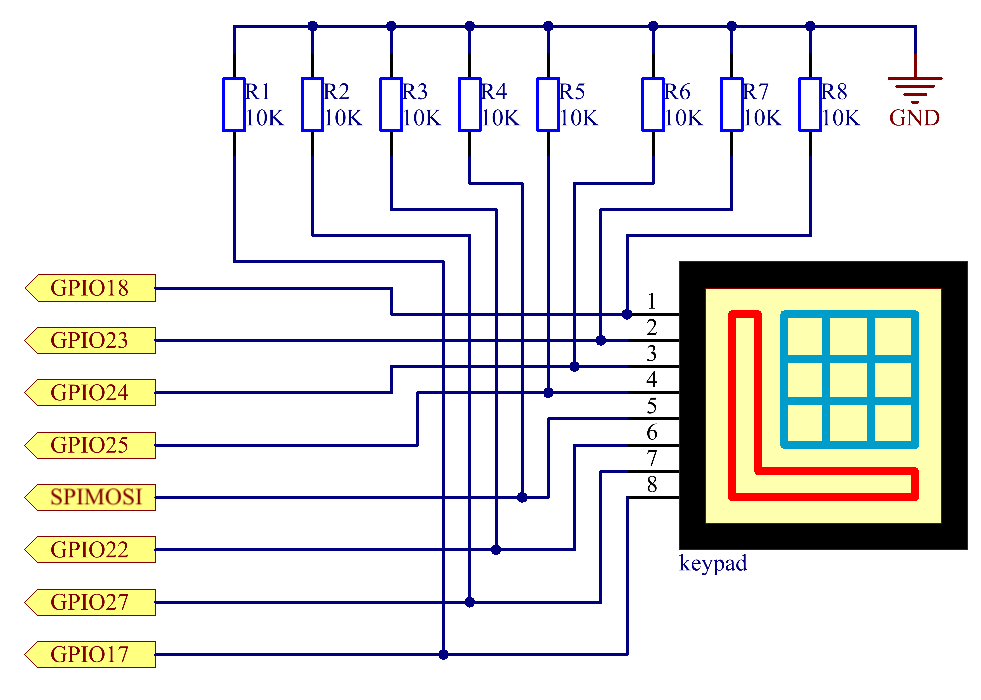## Experimental Procedures¶

Step 1: Build the circuit.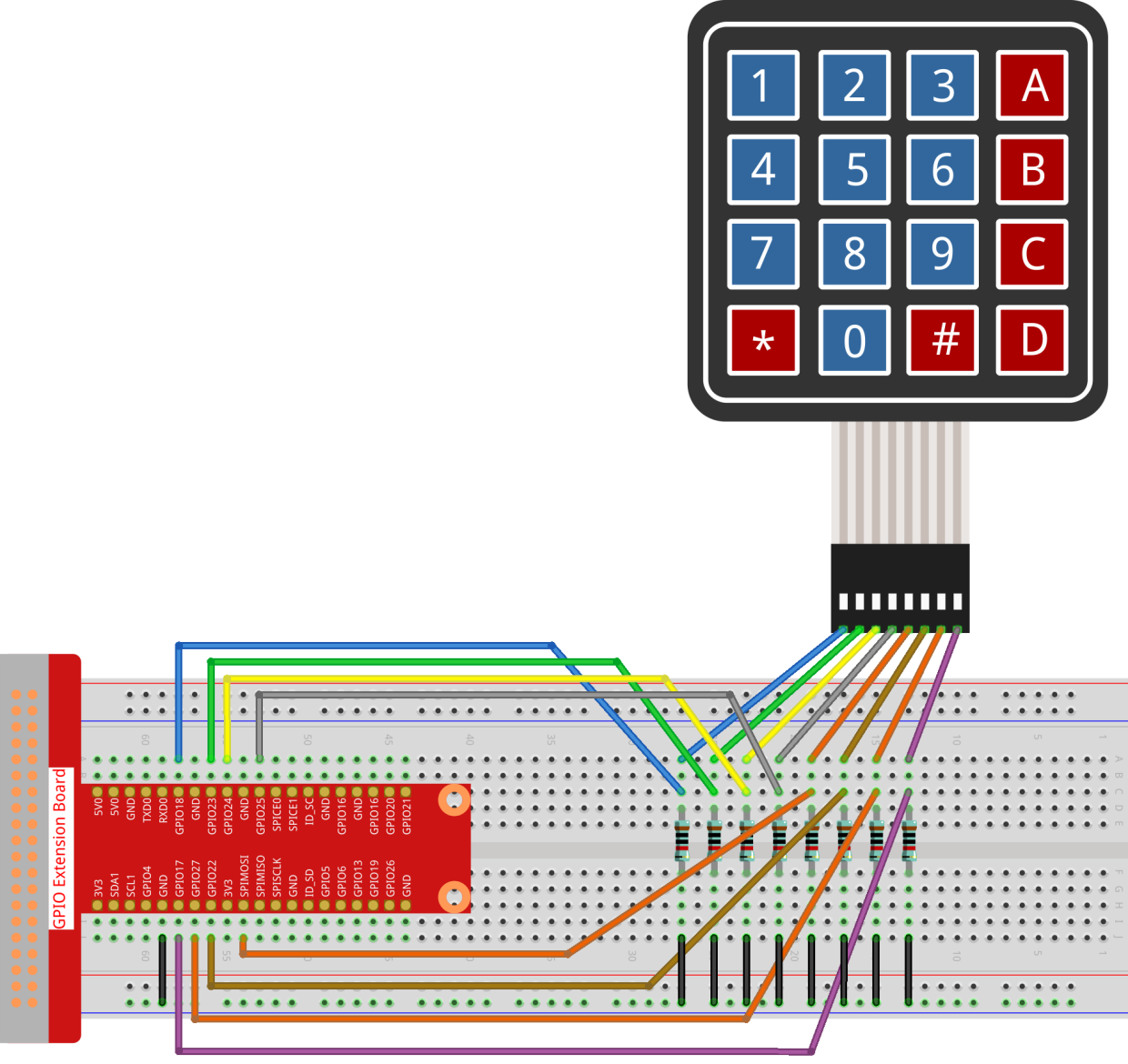### For C Language Users¶

Step 2: Open the code file.

```cd ~/davinci-kit-for-raspberry-pi/c/2.1.5/
```

Step 3: Compile the code.

```gcc 2.1.5_Keypad.cpp -lwiringPi
```

Step 4: Run.

```sudo ./a.out
```

After the code runs, the values of pressed buttons on keypad (button Value) will be printed on the screen.

Note

If it does not work after running, or there is an error prompt: "wiringPi.h: No such file or directory", please refer to C code is not working?.

Code

```#include <wiringPi.h>
#include <stdio.h>

#define ROWS  4
#define COLS  4
#define BUTTON_NUM (ROWS * COLS)

unsigned char KEYS[BUTTON_NUM] {
'1','2','3','A',
'4','5','6','B',
'7','8','9','C',
'*','0','#','D'};

unsigned char rowPins[ROWS] = {1, 4, 5, 6};
unsigned char colPins[COLS] = {12, 3, 2, 0};

bool keyCompare(unsigned char* a, unsigned char* b);
void keyCopy(unsigned char* a, unsigned char* b);
void keyPrint(unsigned char* a);
void keyClear(unsigned char* a);
int keyIndexOf(const char value);

void init(void) {
for(int i=0 ; i<4 ; i++) {
pinMode(rowPins[i], OUTPUT);
pinMode(colPins[i], INPUT);
}
}

int main(void){
unsigned char pressed_keys[BUTTON_NUM];
unsigned char last_key_pressed[BUTTON_NUM];

if(wiringPiSetup() == -1){ //when initialize wiring failed,print message to screen
printf("setup wiringPi failed !");
return 1;
}
init();
while(1){
bool comp = keyCompare(pressed_keys, last_key_pressed);
if (!comp){
keyPrint(pressed_keys);
keyCopy(last_key_pressed, pressed_keys);
}
delay(100);
}
return 0;
}

int index;
int count = 0;
keyClear(result);
for(int i=0 ; i<ROWS ; i++ ){
digitalWrite(rowPins[i], HIGH);
for(int j =0 ; j < COLS ; j++){
index = i * ROWS + j;
result[count]=KEYS[index];
count += 1;
}
}
delay(1);
digitalWrite(rowPins[i], LOW);
}
}

bool keyCompare(unsigned char* a, unsigned char* b){
for (int i=0; i<BUTTON_NUM; i++){
if (a[i] != b[i]){
return false;
}
}
return true;
}

void keyCopy(unsigned char* a, unsigned char* b){
for (int i=0; i<BUTTON_NUM; i++){
a[i] = b[i];
}
}

void keyPrint(unsigned char* a){
if (a != 0){
printf("%c",a);
}
for (int i=1; i<BUTTON_NUM; i++){
if (a[i] != 0){
printf(", %c",a[i]);
}
}
printf("\n");
}

void keyClear(unsigned char* a){
for (int i=0; i<BUTTON_NUM; i++){
a[i] = 0;
}
}

int keyIndexOf(const char value){
for (int i=0; i<BUTTON_NUM; i++){
if ((const char)KEYS[i] == value){
return i;
}
}
return -1;
}
```

Code Explanation

```unsigned char KEYS[BUTTON_NUM] {
'1','2','3','A',
'4','5','6','B',
'7','8','9','C',
'*','0','#','D'};

unsigned char rowPins[ROWS] = {1, 4, 5, 6};
unsigned char colPins[COLS] = {12, 3, 2, 0};
```

Declare each key of the matrix keyboard to the array keys[] and define the pins on each row and column.

```while(1){
bool comp = keyCompare(pressed_keys, last_key_pressed);
if (!comp){
keyPrint(pressed_keys);
keyCopy(last_key_pressed, pressed_keys);
}
delay(100);
}
```

This is the part of the main function that reads and prints the button value.

KeyCompare() and keyCopy() are used to judge whether the state of a button has changed (that is, a button has been pressed or released).

keyPrint() will print the button value of the button whose current level is high level (the button is pressed).

```void keyRead(unsigned char* result){
int index;
int count = 0;
keyClear(result);
for(int i=0 ; i<ROWS ; i++ ){
digitalWrite(rowPins[i], HIGH);
for(int j =0 ; j < COLS ; j++){
index = i * ROWS + j;
result[count]=KEYS[index];
count += 1;
}
}
delay(1);
digitalWrite(rowPins[i], LOW);
}
}
```

This function assigns a high level to each row in turn, and when the key in the column is pressed, the column in which the key is located gets a high level. After the two-layer loop judgment, the key state compilation will generate an array (reasult[]).

When pressing button 3: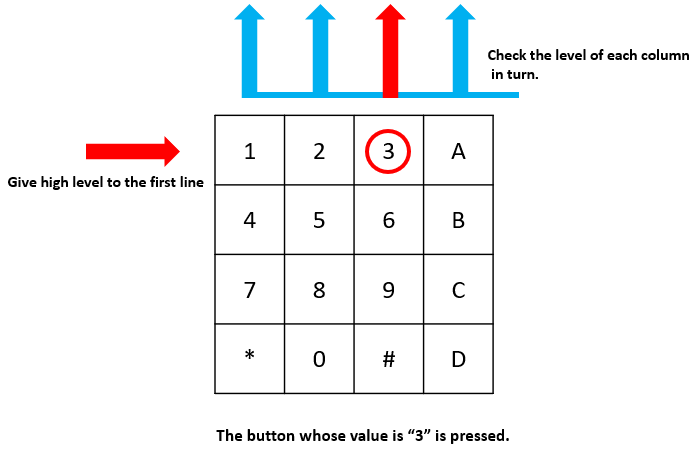RowPin  writes in the high level, and colPin gets the high level. ColPin , colPin, colPin get the low level.

This gives us 0,0,1,0. When rowPin, rowPin and rowPin are written in high level, colPin~colPin will get low level.

After the loop judgment is completed, an array will be generated:

```result[BUTTON_NUM] {
0, 0, 1, 0,
0, 0, 0, 0,
0, 0, 0, 0,
0, 0, 0, 0};
```
```bool keyCompare(unsigned char* a, unsigned char* b){
for (int i=0; i<BUTTON_NUM; i++){
if (a[i] != b[i]){
return false;
}
}
return true;
}

void keyCopy(unsigned char* a, unsigned char* b){
for (int i=0; i<BUTTON_NUM; i++){
a[i] = b[i];
}
}
```

These two functions are used to judge whether the key state has changed, for example when you release your hand when pressing '3' or pressing '2', keyCompare() returns false.

KeyCopy() is used to re-write the current button value for the a array (last_key_pressed[BUTTON_NUM]) after each comparison. So we can compare them next time.

```void keyPrint(unsigned char* a){
//printf("{");
if (a != 0){
printf("%c",a);
}
for (int i=1; i<BUTTON_NUM; i++){
if (a[i] != 0){
printf(", %c",a[i]);
}
}
printf("\n");
}
```

This function is used to print the value of the button currently pressed. If the button '1' is pressed, the '1' will be printed. If the button '1' is pressed and the button '3' is pressed, the '1, 3' will be printed.

### For Python Language Users¶

Step 2: Open the code file.

```cd ~/davinci-kit-for-raspberry-pi/python/
```

Step 3: Run.

```sudo python3 2.1.5_Keypad.py
```

After the code runs, the values of pressed buttons on keypad (button Value) will be printed on the screen.

Code

Note

You can Modify/Reset/Copy/Run/Stop the code below. But before that, you need to go to source code path like `davinci-kit-for-raspberry-pi/python`.

```import RPi.GPIO as GPIO
import time

def __init__(self, rowsPins, colsPins, keys):
self.rowsPins = rowsPins
self.colsPins = colsPins
self.keys = keys
GPIO.setwarnings(False)
GPIO.setmode(GPIO.BCM)
GPIO.setup(self.rowsPins, GPIO.OUT, initial=GPIO.LOW)
GPIO.setup(self.colsPins, GPIO.IN, pull_up_down=GPIO.PUD_DOWN)

pressed_keys = []
for i, row in enumerate(self.rowsPins):
GPIO.output(row, GPIO.HIGH)
for j, col in enumerate(self.colsPins):
index = i * len(self.colsPins) + j
if (GPIO.input(col) == 1):
pressed_keys.append(self.keys[index])
GPIO.output(row, GPIO.LOW)
return pressed_keys

def setup():
rowsPins = [18,23,24,25]
colsPins = [10,22,27,17]
keys = ["1","2","3","A",
"4","5","6","B",
"7","8","9","C",
"*","0","#","D"]
last_key_pressed = []

def loop():
if len(pressed_keys) != 0 and last_key_pressed != pressed_keys:
print(pressed_keys)
last_key_pressed = pressed_keys
time.sleep(0.1)

# Define a destroy function for clean up everything after the script finished
def destroy():
# Release resource
GPIO.cleanup()

if __name__ == '__main__':     # Program start from here
try:
setup()
while True:
loop()
except KeyboardInterrupt:   # When 'Ctrl+C' is pressed, the program destroy() will be executed.
destroy()
```

Code Explanation

```def setup():
rowsPins = [18,23,24,25]
colsPins = [10,22,27,17]
keys = ["1","2","3","A",
"4","5","6","B",
"7","8","9","C",
"*","0","#","D"]
last_key_pressed = []
```

Declare each key of the matrix keyboard to the array keys[] and define the pins on each row and column.

```def loop():
if len(pressed_keys) != 0 and last_key_pressed != pressed_keys:
print(pressed_keys)
last_key_pressed = pressed_keys
time.sleep(0.1)
```

This is the part of the main function that reads and prints the button value.

The statement if len(pressed_keys) != 0 and last_key_pressed != pressed_keys is used to judge

whether a key is pressed and the state of the pressed button. (If you press '3' when you press '1', the judgement is tenable.)

Prints the value of the currently pressed key when the condition is tenable.

The statement last_key_pressed = pressed_keys assigns the state of each judgment to an array last_key_pressed to facilitate the next round of conditional judgment.

```def read(self):
pressed_keys = []
for i, row in enumerate(self.rowsPins):
GPIO.output(row, GPIO.HIGH)
for j, col in enumerate(self.colsPins):
index = i * len(self.colsPins) + j
if (GPIO.input(col) == 1):
pressed_keys.append(self.keys[index])
GPIO.output(row, GPIO.LOW)
return pressed_keys
```

This function assigns a high level to each row in turn, and when the button in the column is pressed, the column in which the key is located gets a high level. After the two-layer loop is judged, the value of the button whose state is 1 is stored in the array pressed_keys.

If you press the key '3':rowPins is written in high level, and colPins gets high level.

colPins、colPins、colPins get low level.

There are four states:0, 0, 1, 0; and we write '3' into pressed_keys.

When rowPins , rowPins , rowPins are written into high level, colPins ~ colPins get low level.

The loop stopped, there returns pressed_keys = '3'.

If you press the buttons '1' and '3', there will return pressed_keys = ['1','3'].

## Phenomenon Picture¶